# NCERT Solutions for Class 8 Maths Chapter 2 Exercise 2.2 – Linear Equation in one variable

NCERT Solutions for Class 8 Maths Chapter 2 Exercise 2.2 – Linear Equation in one variable, has been designed by the NCERT to test the knowledge of the student on the topic – Some Applications of Linear Equation in one variable

### NCERT Solutions for Class 8 Maths Chapter 2 Exercise 2.2 – Linear Equation in one variable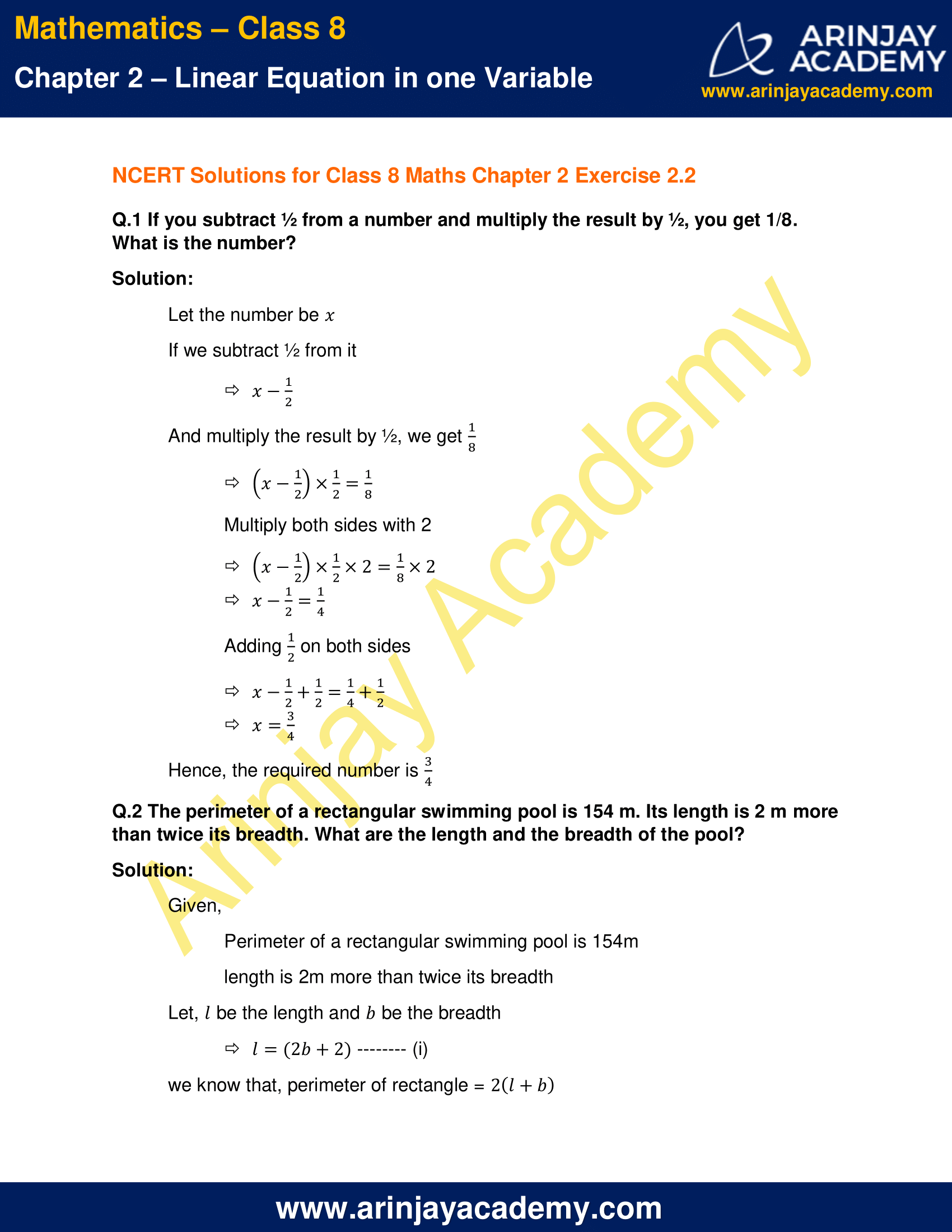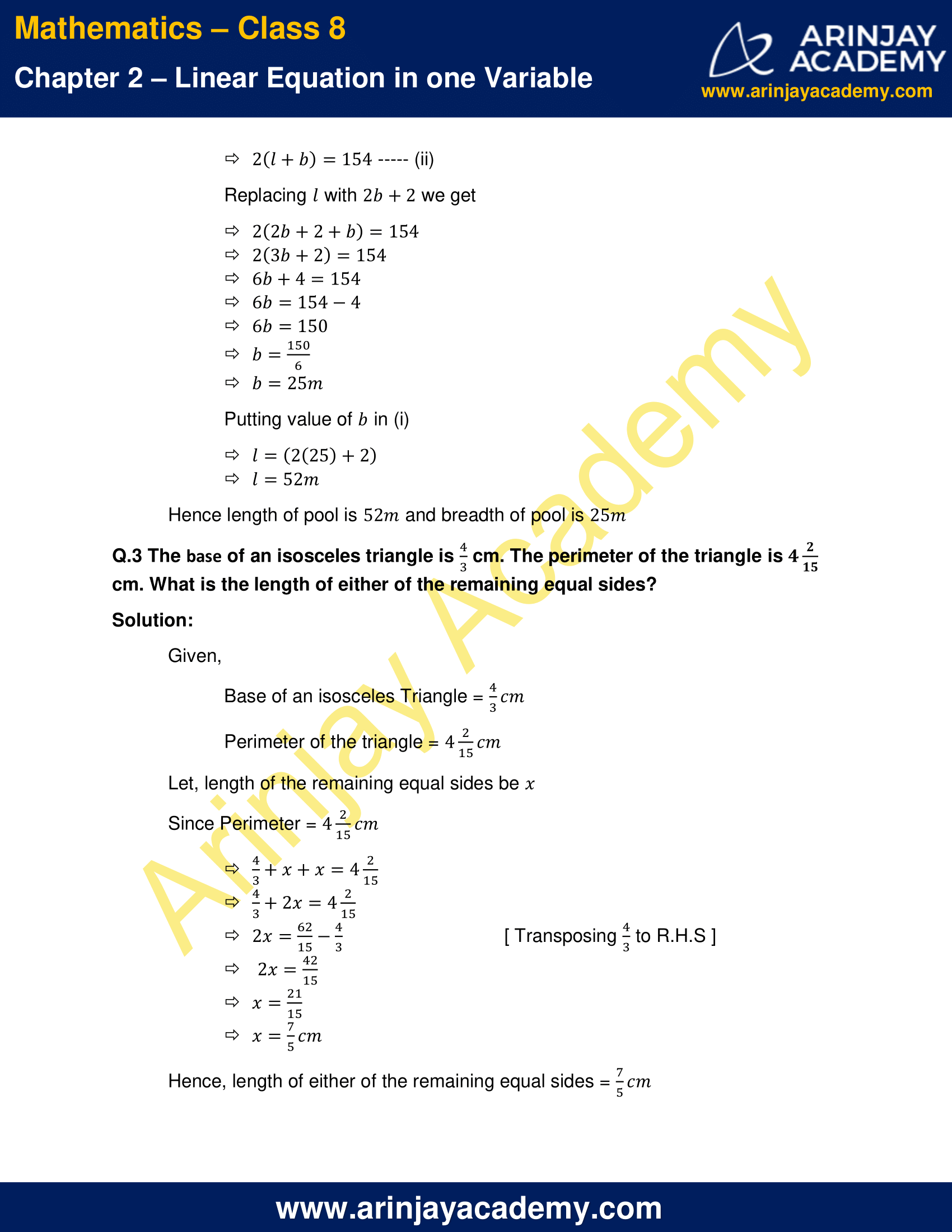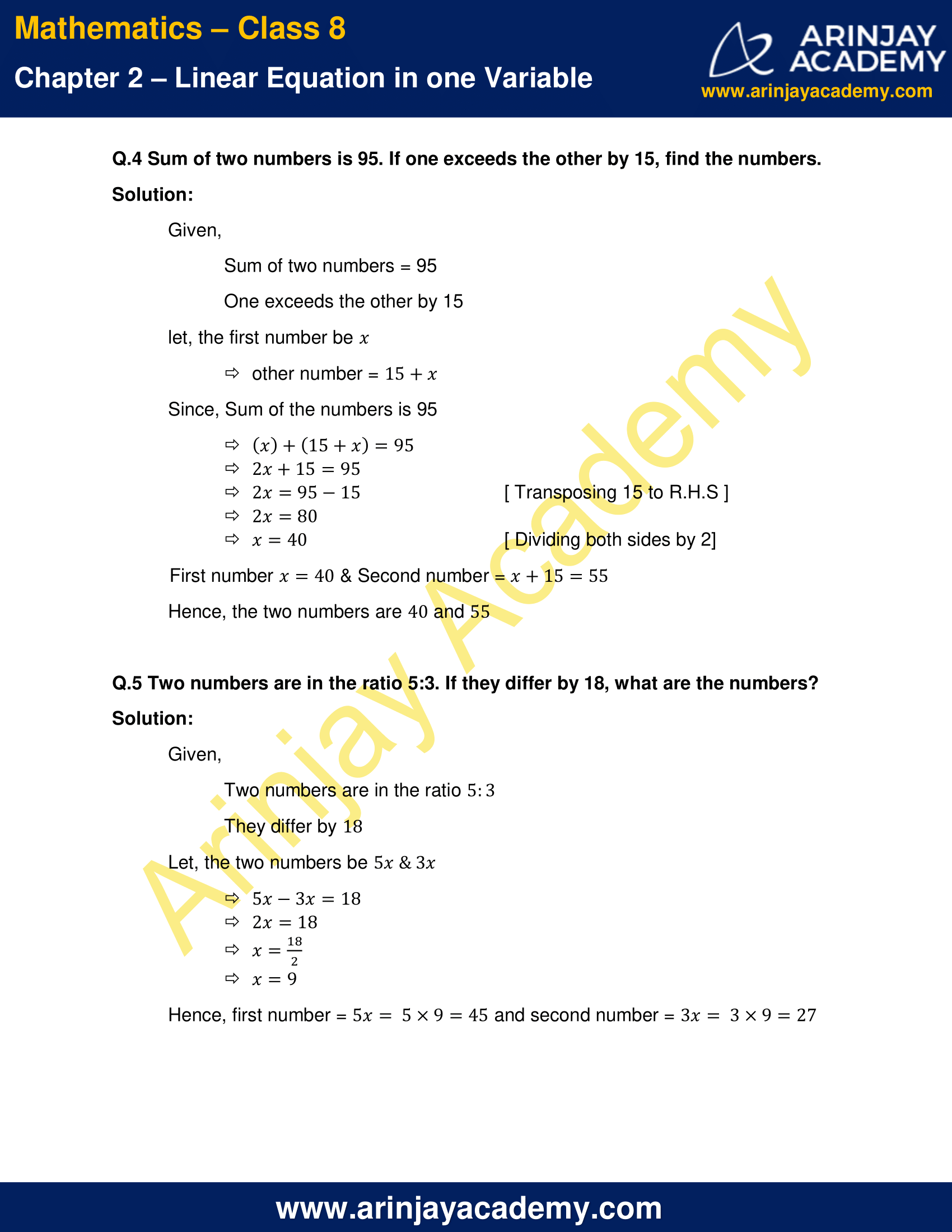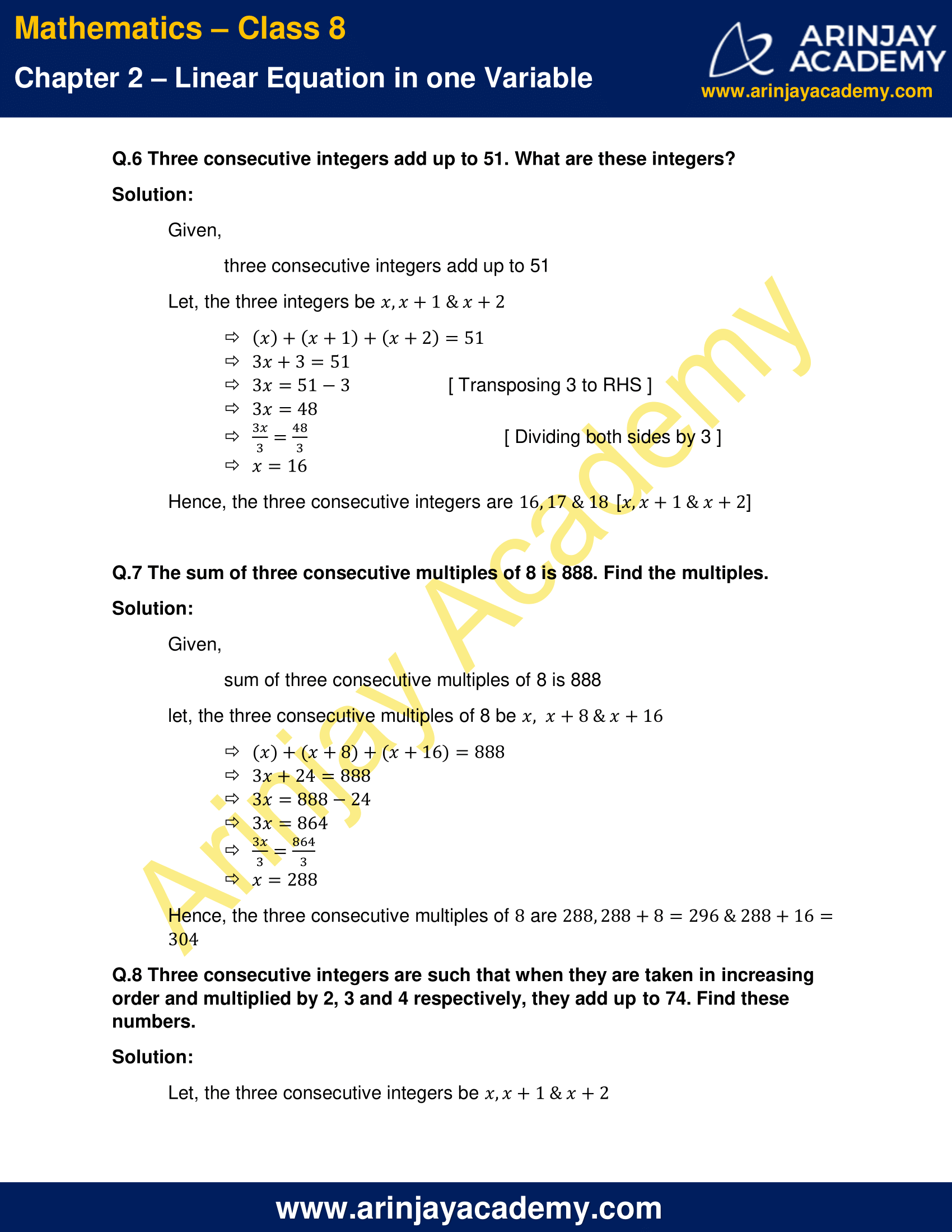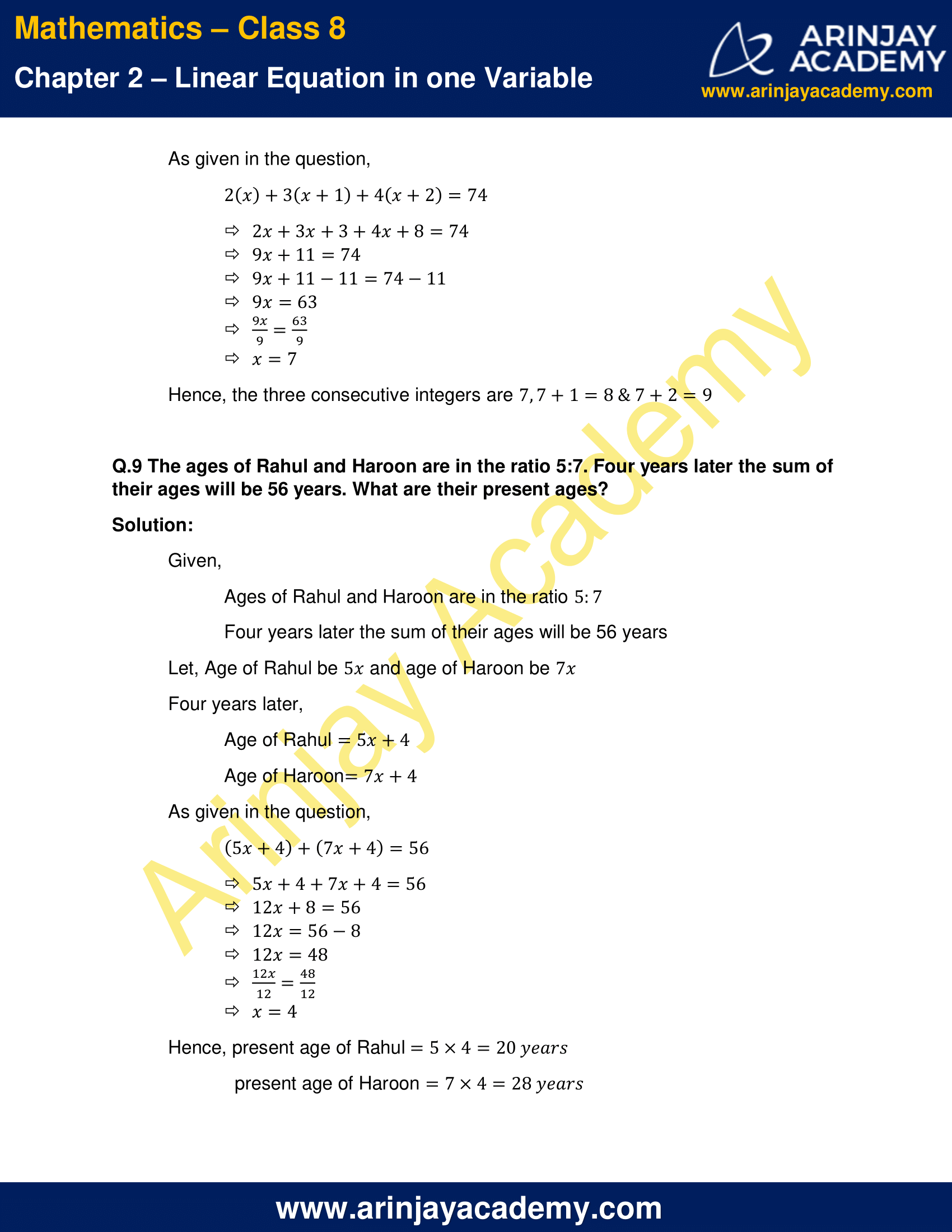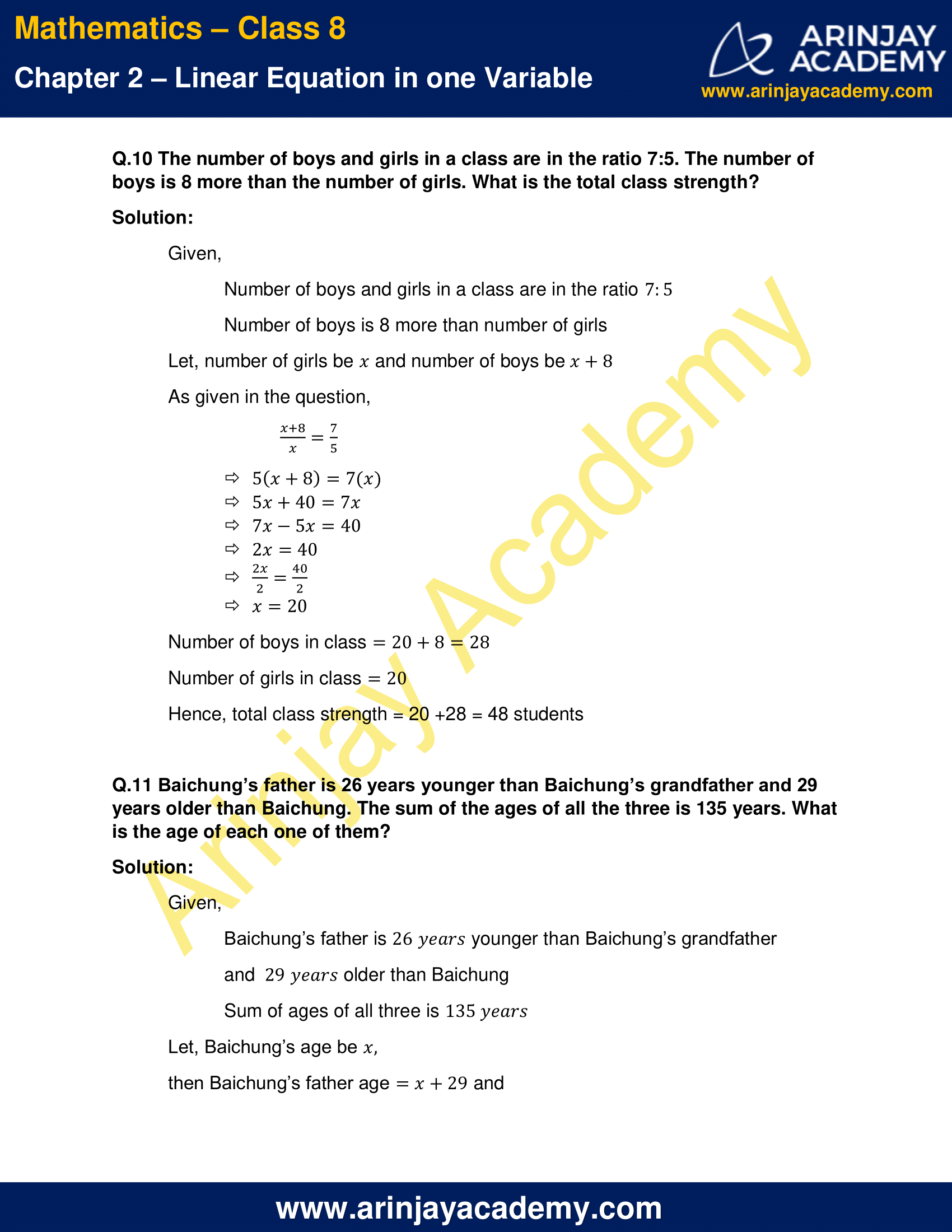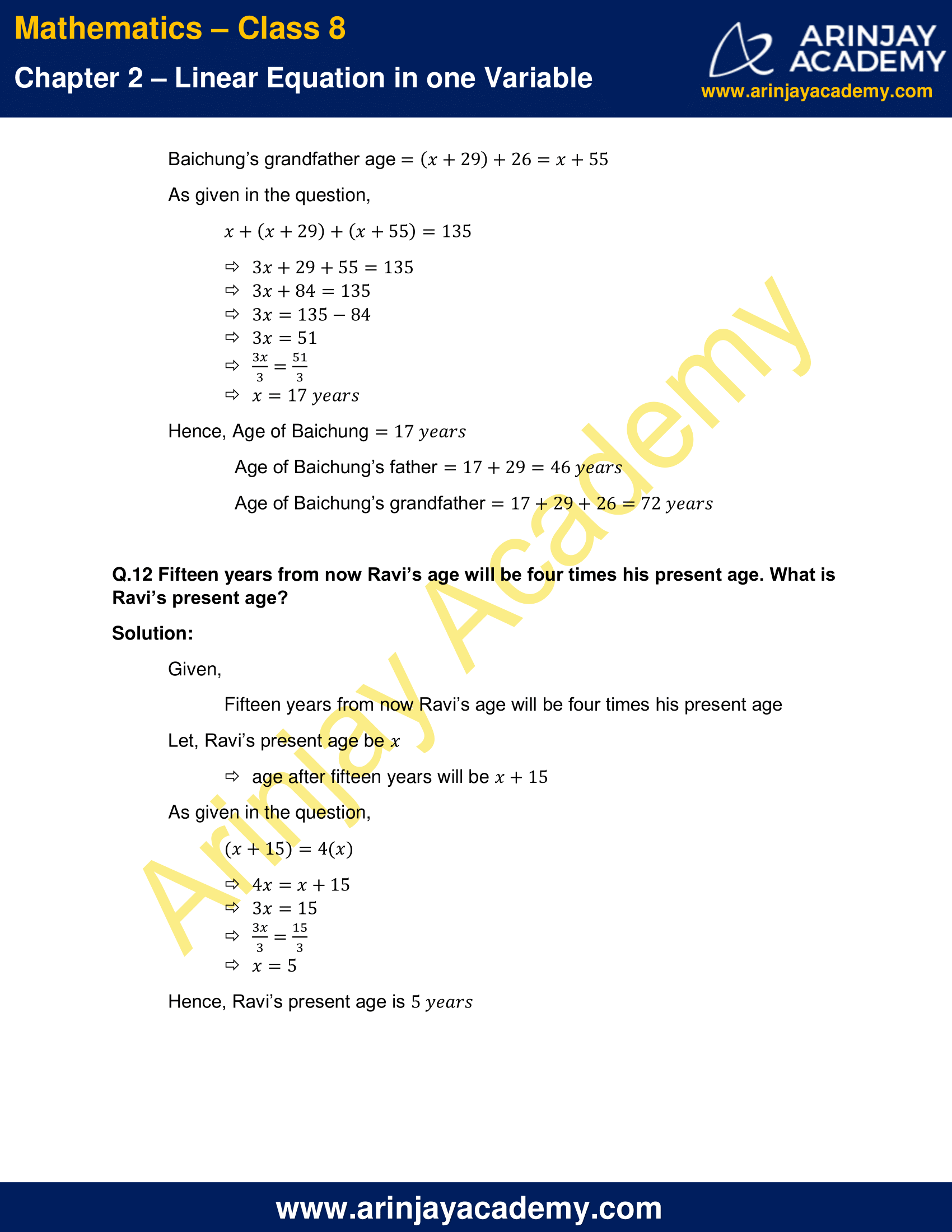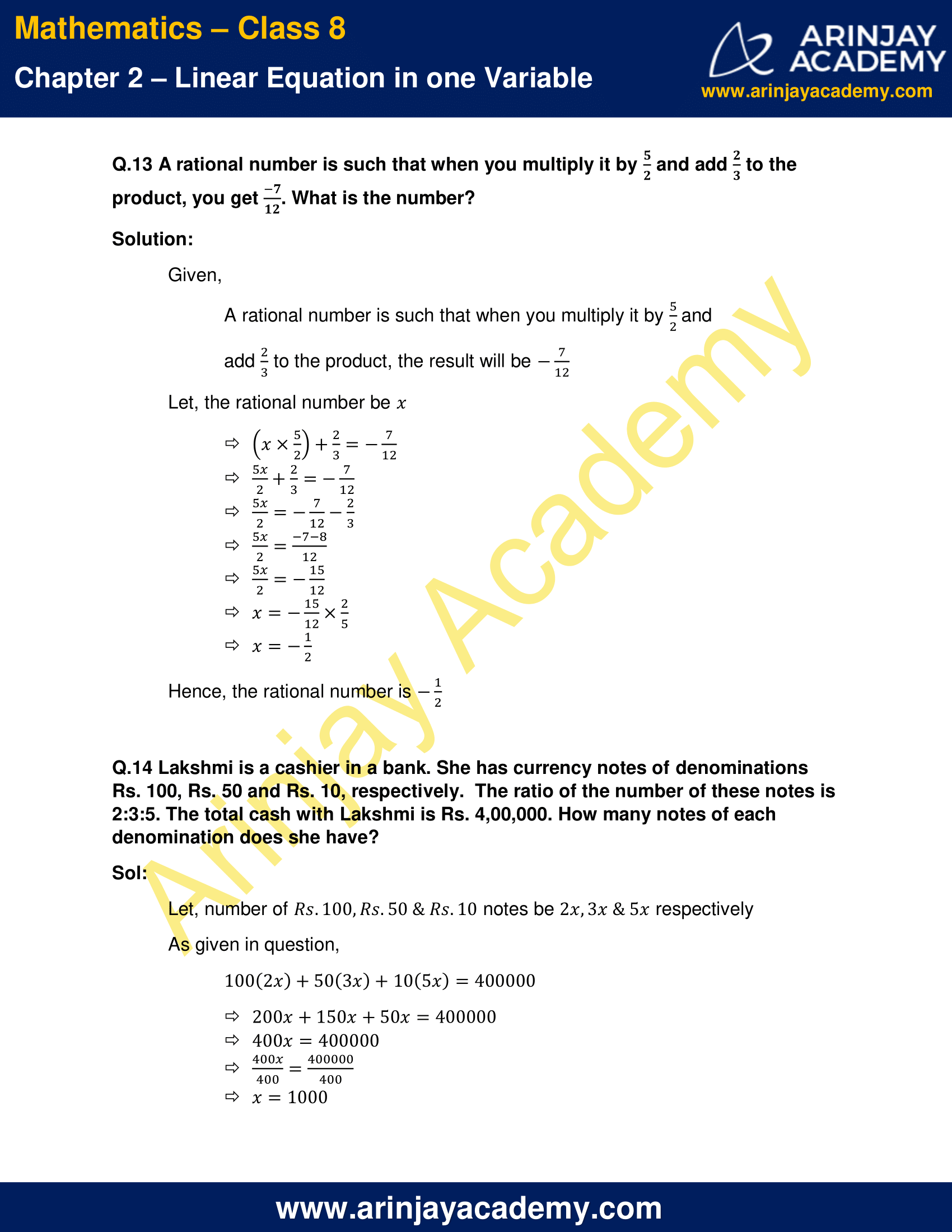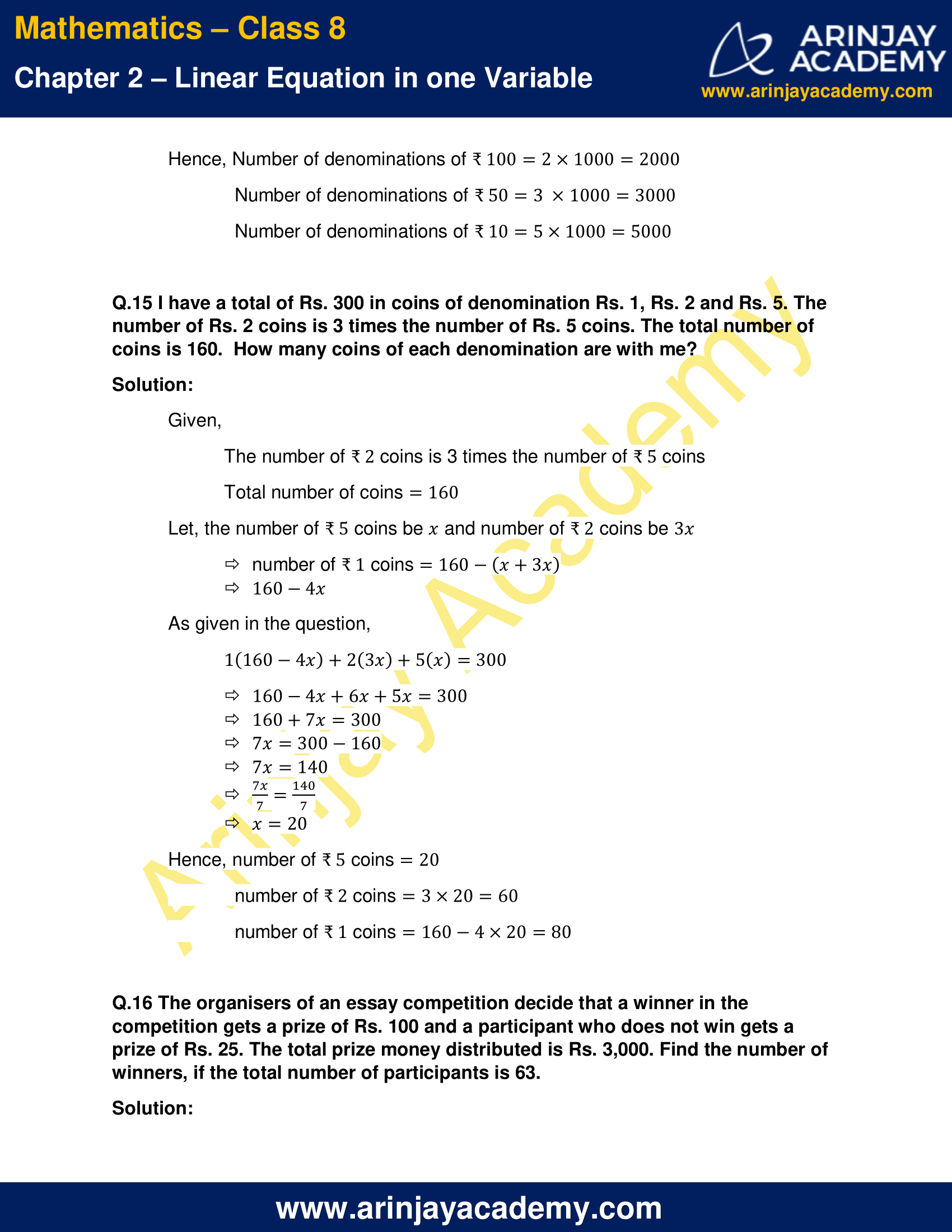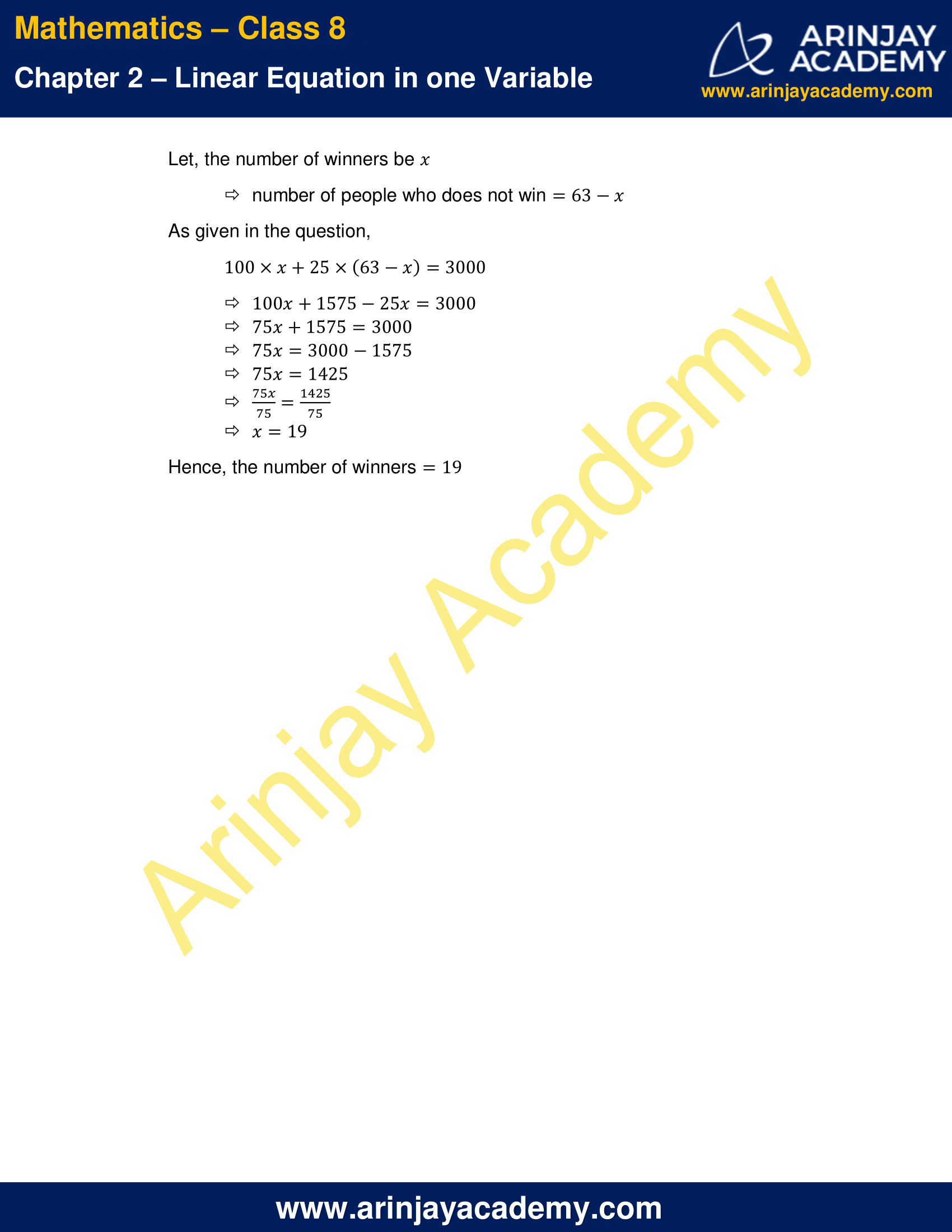NCERT Solutions for Class 8 Maths Chapter 2 Exercise 2.2 – Linear Equation in one variable

Q.1 If you subtract (1/2) from a number and multiply the result by (1/2), you get (1/8). What is the number?

Solution:

Let the number be ‘x’

If we subtract (1/2) from it

x – (1/2)

And multiply the result by (1/2), we get (1/8)

[x – (1/2)] × (1/2) = (1/8)

Multiply both sides with 2

[x – (1/2)] × (1/2) × 2 = (1/8) × 2

x – (1/2) = (1/4)

x – (1/2) + (1/2) = (1/4) + (1/2)

x = (3/4)

Hence, the required number is (3/4)

Q.2 The perimeter of a rectangular swimming pool is 154 m. Its length is 2 m more than twice its breadth. What are the length and the breadth of the pool?

Solution:

Given,

Perimeter of a rectangular swimming pool is 154m

length is 2m more than twice its breadth

Let, l be the length and b be the breadth

l=(2b+2) ——– (i)

we know that, perimeter of rectangle = 2(l+b)

2(l+b)=154—–(ii)

Replacing l with 2b+2 we get

2(2b+2+b)=154

2(3b+2)=154

6b+4=154

6b=154-4

6b=150

b=(150/6)

b=25m

Putting value of b in (i)

l=(2(25)+2)

l=52m

Hence length of pool is 52m and breadth of pool is 25m.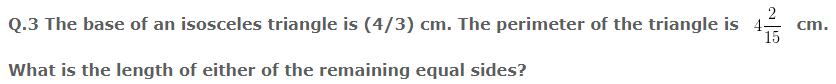Solution:

Given,

Base of an isosceles Triangle = (4/3) cm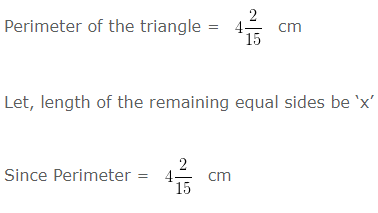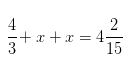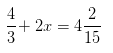2x = (62/15) – (4/3) [ Transposing (4/3) to R.H.S ]

2x = 42/15

x = 21/15

x = (7/5) cm

Hence, length of either of the remaining equal sides = (7/5) cm

Q.4 Sum of two numbers is 95. If one exceeds the other by 15, find the numbers.

Solution:

Given,

Sum of two numbers = 95

One exceeds the other by 15

let, the first number be ‘x’

other number = 15+x

Since, Sum of the numbers is 95

(x)+(15+x)=95

2x+15=95

2x=95-15     [Transposing 15 to R.H.S]

2x=80

x=40    [ Dividing both sides by 2]

First number x = 40  & Second number = x + 15 = 55

Hence, the two numbers are 40 and 55

Q.5 Two numbers are in the ratio 5:3. If they differ by 18, what are the numbers?

Solution:

Given,

Two numbers are in the ratio 5:3

They differ by 18

Let, the two numbers be 5x & 3x

5x-3x=18

2x=18

x= 18/2

x=9

Hence, first number = 5x = 5 × 9=45 and second number = 3x = 3 × 9 = 27

Q.6 Three consecutive integers add up to 51. What are these integers?

Solution:

Given,

three consecutive integers add up to 51

Let, the three integers be x, x+1 and x+2

(x)+(x+1)+(x+2)=51

3x+3=51

3x=51-3  [ Transposing 3 to RHS ]

3x=48

3x/3 = 48/3  [ Dividing both sides by 3 ]

x = 16

Hence, the three consecutive integers are 16, 17, 18 [x, x+1 and x+2]

Q.7 The sum of three consecutive multiples of 8 is 888. Find the multiples.

Solution:

Given,

Sum of three consecutive multiples of 8 is 888

Let, the three consecutive multiples of 8 be (x, x+8 and x+16)

(x)+(x+8)+(x+16)=888

3x+24=888

3x=888-24

3x=864

3x/3 = (864/3)

x = 288

Hence, the three consecutive multiples of 8 are (288, 288+8=296 and 288+16=304)

Q.8 Three consecutive integers are such that when they are taken in increasing order and multiplied by 2, 3 and 4 respectively, they add up to 74. Find these numbers.

Solution:

Let, the three consecutive integers be (x, x+1 and x+2)

As given in the question,

2(x)+3(x+1)+4(x+2)=74

2x+3x+3+4x+8=74

9x+11=74[/latex]

9x+11-11=74-11[/latex]

9x=63[/latex]

9x/9 = 63/9

x = 7

Hence, the three consecutive integers are 7, 7+1=8 & 7+2=9

Q.9 The ages of Rahul and Haroon are in the ratio 5:7. Four years later the sum of their ages will be 56 years. What are their present ages?

Solution:

Given,

Ages of Rahul and Haroon are in the ratio 5:7

Four years later the sum of their ages will be 56 years

Let, Age of Rahul be 5x and age of Haroon be 7x

Four years later,

Age of Rahul = 5x+4

Age of Haroon = 7x+4

As given in the question,

(5x+4) + (7x+4) = 56

5x+4+7x+4=56

12x+8=56

12x=56-8

12x=48

12x/12 = 48/12

x=4

Hence, present age of Rahul = 5 x 4 = 20 years

present age of Haroon = 7 x 4 = 28 years

Q.10 The number of boys and girls in a class are in the ratio 7:5. The number of boys is 8 more than the number of girls. What is the total class strength?

Solution:

Given,

Number of boys and girls in a class are in the ratio 7:5

Number of boys is 8 more than number of girls

Let, number of girls be ‘x’ and number of boys be ‘x+8’

As given in the question,

x+8/x = 7/5

5(x+8)=7(x)

5x+40=7x

7x-5x=40

2x=40

2x/2 = 40/2

x=20

Number of boys in class = 20+8 = 28

Number of girls in class = 20

Hence, total class strength = 20 +28 = 48 students

Q.11 Baichung’s father is 26 years younger than Baichung’s grandfather and 29 years older than Baichung. The sum of the ages of all the three is 135 years. What is the age of each one of them?

Solution:

Given,

Baichung’s father is 26 years younger than Baichung’s grandfather and 29 years older than Baichung

Sum of ages of all three is 135 years

Let, Baichung’s age be x

then Baichung’s father age x+29 and

Baichung’s grandfather age = (x+29) + 26 = x+55

As given in the question,

x+(x+29)+(x+55)=135

3x+29+55=135

3x+84=135

3x=135-84

3x=51

(3x/3) = (51/3)

x = 17years

Hence, Age of Baichung = 17 years

Age of Baichung’s father = 17 + 29 = 46 years

Age of Baichung’s grandfather = 17 + 29 + 26 = 72 years

Q.12 Fifteen years from now Ravi’s age will be four times his present age. What is Ravi’s present age?

Solution:

Given,

Fifteen years from now Ravi’s age will be four times his present age

Let, Ravi’s present age be x

age after fifteen years will be x+15

As given in the question,

(x+15)=4(x)

4x=x+15

3x=15

(3x/3) = (15/3)

x=5

Hence, Ravi’s present age is 5 years

Q.13 A rational number is such that when you multiply it by (5/2) and add (2/3) to the product, you get (-7/12) . What is the number?

Solution:

Given,

A rational number is such that when you multiply it by (5/2) and add (2/3) to the product, the result will be (-7/12)

Let, the rational number be x

[x × (5/2)]+ (2/3) = (-7/12)

(5x/2) + (2/3) = (-7/12)

(5x)/2 = (-7/12) – (2/3)

5x/2 = (-7-8)/12

5x/2 = (-15/12)

x = (-15/12) × (2/5)

x = (-1/2)

Hence, the rational number is (-1/2)

Q.14 Lakshmi is a cashier in a bank. She has currency notes of denominations Rs. 100, Rs. 50 and Rs. 10, respectively.  The ratio of the number of these notes is 2:3:5. The total cash with Lakshmi is Rs. 4,00,000. How many notes of each denomination does she have?

Sol:

Let, number of Rs.100, Rs.50 & Rs.10 notes be 2x, 3x and 5x respectively

As given in question,

100(2x)+50(3x)+10(5x)=400000

200x+150x+50x=400000

400x=400000

(400x/400) = (400000/400)

x=1000

Hence, Number of denominations of ₹100=2×1000=2000

Number of denominations of ₹ 50=3 ×1000=3000

Number of denominations of ₹ 10=5×1000=5000

Q.15 I have a total of Rs. 300 in coins of denomination Rs. 1, Rs. 2 and Rs. 5. The number of Rs. 2 coins is 3 times the number of Rs. 5 coins. The total number of coins is 160.  How many coins of each denomination are with me?

Solution:

Given,

The number of ₹ 2 coins is 3 times the number of ₹ 5 coins

Total number of coins = 160

Let, the number of ₹ 5 coins be x and number of ₹ 2 coins be 3x

number of ₹ 1 coins = 160 – (x+3x)

= 160-4x

As given in the question,

1(160-4x)+2(3x)+5(x)=300

160-4x+6x+5x=300

160+7x=300

7x=300-160

7x=140

(7x/7) = (140/7)

x=20

Hence, number of ₹ 5 coins = 20

number of ₹ 2 coins = 3 x 20 = 60

number of ₹ 1 coins = 160 – 4 × 20 = 80

Q.16 The organisers of an essay competition decide that a winner in the competition gets a prize of Rs. 100 and a participant who does not win gets a prize of Rs. 25. The total prize money distributed is Rs. 3,000. Find the number of winners, if the total number of participants is 63.

Solution:

Let, the number of winners be x

number of people who does not win 63 – x

As given in the question,

100×x+25×(63-x)=3000

100x+1575-25x=3000

75x+1575=3000

75x=3000-1575

75x=1425

(75x/75) = (1425/75)

x=19

Hence, the number of winners = 19

The next Exercise for NCERT Solutions for Class 8 Maths Chapter 2 Exercise 2.3 – Linear Equation in one variable can be accessed by clicking here